Example problem: Adaptive solution of the 3D Poisson equation in a spherical domain

Following the numerous 2D problems discussed in earlier examples we now demonstrate that the solution of 3D problems is just as easy. For this purpose we discuss the adaptive solution of the 3D Poisson problem

 Three-dimensional model Poisson problem Solve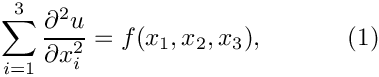in the "eighth-of-a-sphere" domain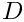, with Dirichlet boundary conditions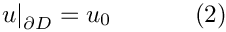where the function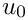is given.

We choose a source function and boundary conditions for which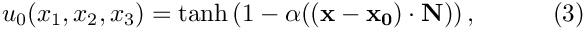is the exact solution. Here where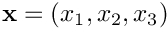is the vector of the spatial coordinates, and the vectors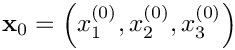and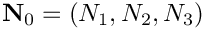are constants. For large values of the constant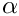the solution varies rapidly across the plane through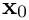whose normal is given by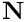.

Here are some plots of the exact and computed solutions for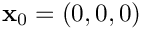,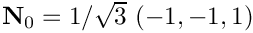, and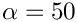at various levels of mesh refinement. Note that the plot of the exact solution was produced by setting the nodal values to the exact solution, obtained by evaluating (3) at the nodal positions. The elements' basis functions were then used to interpolate between the nodal values. On the coarse meshes, the interpolation between the "exact" nodal values is clearly inadequate to resolve the rapid variation of the solution.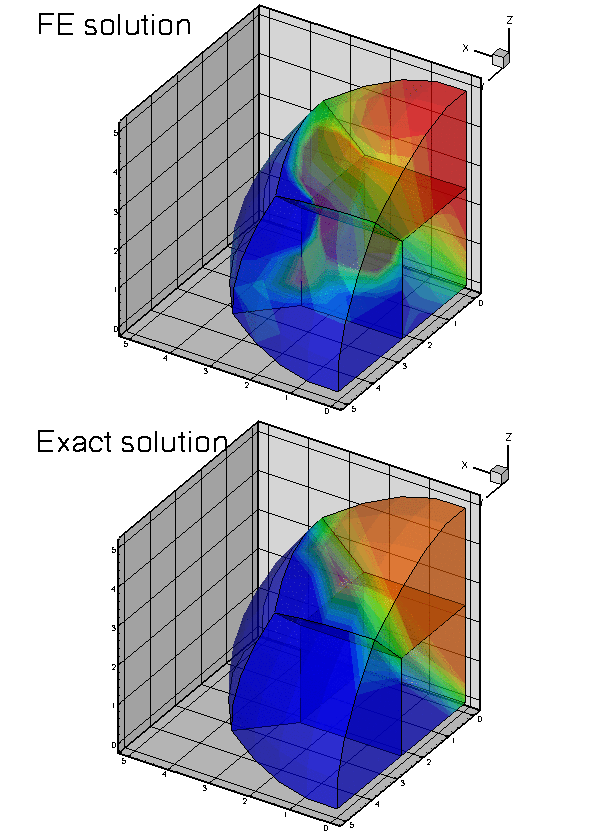Plot of the solution

# Global parameters and functions

Following our usual practice, we use a namespace, TanhSolnForPoisson, to define the source function, the exact solution and various problem parameters.

//=============start_of_namespace=====================================
/// Namespace for exact solution for Poisson equation with sharp step
//====================================================================
{
/// Parameter for steepness of step
double Alpha=1;
/// Orientation (non-normalised x-component of unit vector in direction
/// of step plane)
double N_x=-1.0;
/// Orientation (non-normalised y-component of unit vector in direction
/// of step plane)
double N_y=-1.0;
/// Orientation (non-normalised z-component of unit vector in direction
/// of step plane)
double N_z=1.0;
/// Orientation (x-coordinate of step plane)
double X_0=0.0;
/// Orientation (y-coordinate of step plane)
double Y_0=0.0;
/// Orientation (z-coordinate of step plane)
double Z_0=0.0;
// Exact solution as a Vector
void get_exact_u(const Vector<double>& x, Vector<double>& u)
{
u = tanh(Alpha*((x-X_0)*N_x/sqrt(N_x*N_x+N_y*N_y+N_z*N_z)+(x-Y_0)*
N_y/sqrt(N_x*N_x+N_y*N_y+N_z*N_z)+(x-Z_0)*
N_z/sqrt(N_x*N_x+N_y*N_y+N_z*N_z)));
}
/// Exact solution as a scalar
void get_exact_u(const Vector<double>& x, double& u)
{
u = tanh(Alpha*((x-X_0)*N_x/sqrt(N_x*N_x+N_y*N_y+N_z*N_z)+(x-Y_0)*
N_y/sqrt(N_x*N_x+N_y*N_y+N_z*N_z)+(x-Z_0)*
N_z/sqrt(N_x*N_x+N_y*N_y+N_z*N_z)));
}
/// Source function to make it an exact solution
void get_source(const Vector<double>& x, double& source)
{
double s1,s2,s3,s4;
s1 = -2.0*tanh(Alpha*((x-X_0)*N_x/sqrt(N_x*N_x+N_y*N_y+N_z*N_z)+(x-
Y_0)*N_y/sqrt(N_x*N_x+N_y*N_y+N_z*N_z)+(x-Z_0)*N_z/sqrt(N_x*N_x+N_y*N_y+N_z*
N_z)))*(1.0-pow(tanh(Alpha*((x-X_0)*N_x/sqrt(N_x*N_x+N_y*N_y+N_z*N_z)+(x-
Y_0)*N_y/sqrt(N_x*N_x+N_y*N_y+N_z*N_z)+(x-Z_0)*N_z/sqrt(N_x*N_x+N_y*N_y+N_z*
s3 = -2.0*tanh(Alpha*((x-X_0)*N_x/sqrt(N_x*N_x+N_y*N_y+N_z*N_z)+(x-
Y_0)*N_y/sqrt(N_x*N_x+N_y*N_y+N_z*N_z)+(x-Z_0)*N_z/sqrt(N_x*N_x+N_y*N_y+N_z*
N_z)))*(1.0-pow(tanh(Alpha*((x-X_0)*N_x/sqrt(N_x*N_x+N_y*N_y+N_z*N_z)+(x-
Y_0)*N_y/sqrt(N_x*N_x+N_y*N_y+N_z*N_z)+(x-Z_0)*N_z/sqrt(N_x*N_x+N_y*N_y+N_z*
s4 = -2.0*tanh(Alpha*((x-X_0)*N_x/sqrt(N_x*N_x+N_y*N_y+N_z*N_z)+(x-
Y_0)*N_y/sqrt(N_x*N_x+N_y*N_y+N_z*N_z)+(x-Z_0)*N_z/sqrt(N_x*N_x+N_y*N_y+N_z*
N_z)))*(1.0-pow(tanh(Alpha*((x-X_0)*N_x/sqrt(N_x*N_x+N_y*N_y+N_z*N_z)+(x-
Y_0)*N_y/sqrt(N_x*N_x+N_y*N_y+N_z*N_z)+(x-Z_0)*N_z/sqrt(N_x*N_x+N_y*N_y+N_z*
s2 = s3+s4;
source = s1+s2;
}
} // end of namespace
Namespace for exact solution for Poisson equation with sharp step.
double N_y
Orientation (non-normalised y-component of unit vector in direction of step plane)
double Z_0
Orientation (z-coordinate of step plane)
double N_x
Orientation (non-normalised x-component of unit vector in direction of step plane)
double Y_0
Orientation (y-coordinate of step plane)
void get_source(const Vector< double > &x, double &source)
Source function to make it an exact solution.
double Alpha
Parameter for steepness of step.
double N_z
Orientation (non-normalised z-component of unit vector in direction of step plane)
double X_0
Orientation (x-coordinate of step plane)
void get_exact_u(const Vector< double > &x, Vector< double > &u)

# The driver code

The driver code solves the 3D Poisson problem with full spatial adaptivity – a fairly time-consuming process. To minimise the run-times when the code is executed during oomph-lib's self-tests, we use command line arguments to optionally limit the number of adaptive refinements. If the code is run with a(ny) command line arguments, only a single adaptive refinement is performed; otherwise up to four levels of refinement are permitted. oomph-lib provides storage for the command line arguments in the namespace CommandLineArgs to make them accessible to other parts of the code.

Otherwise the driver code is very similar to that used in the corresponding 2D Poisson problems: We construct the problem, passing the pointer to the source function. Next, we create a DocInfo object to specify the output directory, and execute the global self-test to assert that the problem has been set up correctly. Next we solve the problem on the coarse initial mesh (comprising four 27-node brick elements) and then adapt the problem based on the elemental error estimates, until the maximum number of adaptations has been reached or until the adaptation ceases to changes the mesh.

//=========start_of_main=============================================
/// Driver for 3D Poisson problem in eighth of a sphere. Solution
/// has a sharp step. If there are
/// any command line arguments, we regard this as a validation run
/// and perform only a single adaptation.
//===================================================================
int main(int argc, char *argv[])
{
// Store command line arguments
CommandLineArgs::setup(argc,argv);
// Set up the problem with 27-node brick elements, pass pointer to
// source function
// Setup labels for output
DocInfo doc_info;
// Output directory
doc_info.set_directory("RESLT");
// Step number
doc_info.number()=0;
// Check if we're ready to go
cout << "Self test: " << problem.self_test() << std::endl;
// Solve the problem
problem.newton_solve();
//Output solution
problem.doc_solution(doc_info);
//Increment counter for solutions
doc_info.number()++;
// Now do (up to) three rounds of fully automatic adapation in response to
// error estimate
unsigned max_solve;
if (CommandLineArgs::Argc>1)
{
// Validation run: Just one adaptation
max_solve=1;
cout << "Only doing one adaptation for validation" << std::endl;
}
else
{
max_solve=4;
}
for (unsigned isolve=0;isolve<max_solve;isolve++)
{
// Re-solve the problem if the adaptation has changed anything
if ((problem.mesh_pt()->nrefined() !=0)||
(problem.mesh_pt()->nunrefined()!=0))
{
problem.newton_solve();
}
else
{
cout << "Mesh wasn't adapted --> we'll stop here" << std::endl;
break;
}
//Output solution
problem.doc_solution(doc_info);
//Increment counter for solutions
doc_info.number()++;
}
// pause("done");
} // end of main
///////////////////////////////////////////////////////////////////// ///////////////////////////////...
int main(int argc, char *argv[])
///////////////////////////////////////////////////////////////////// ///////////////////////////////...

# The problem class

The problem class has the usual structure – the only difference to the corresponding 2D codes is that the assignment of the boundary conditions in actions_before_newton_solve() now involves thee nodal coordinates rather than two.

//=======start_of_class_definition====================================
/// Poisson problem in refineable eighth of a sphere mesh.
//====================================================================
template<class ELEMENT>
class EighthSpherePoissonProblem : public Problem
{
public:
/// Constructor: Pass pointer to source function
PoissonEquations<3>::PoissonSourceFctPt source_fct_pt);
/// Destructor: Empty
/// Overload generic access function by one that returns
/// a pointer to the specific mesh
RefineableEighthSphereMesh<ELEMENT>* mesh_pt()
{
return dynamic_cast<RefineableEighthSphereMesh<ELEMENT>*>(Problem::mesh_pt());
}
/// Update the problem specs after solve (empty)
/// Update the problem specs before solve:
/// Set Dirchlet boundary conditions from exact solution.
{
//Loop over the boundaries
unsigned num_bound = mesh_pt()->nboundary();
for(unsigned ibound=0;ibound<num_bound;ibound++)
{
// Loop over the nodes on boundary
unsigned num_nod=mesh_pt()->nboundary_node(ibound);
for (unsigned inod=0;inod<num_nod;inod++)
{
Node* nod_pt=mesh_pt()->boundary_node_pt(ibound,inod);
double u;
Vector<double> x(3);
x=nod_pt->x(0);
x=nod_pt->x(1);
x=nod_pt->x(2);
nod_pt->set_value(0,u);
}
}
}
/// Doc the solution
void doc_solution(DocInfo& doc_info);
private:
/// Pointer to source function
PoissonEquations<3>::PoissonSourceFctPt Source_fct_pt;
}; // end of class definition
~EighthSpherePoissonProblem()
Destructor: Empty.
void actions_after_newton_solve()
Update the problem specs after solve (empty)
RefineableEighthSphereMesh< ELEMENT > * mesh_pt()
Overload generic access function by one that returns a pointer to the specific mesh.
void doc_solution(DocInfo &doc_info)
Doc the solution.
EighthSpherePoissonProblem(PoissonEquations< 3 >::PoissonSourceFctPt source_fct_pt)
Constructor: Pass pointer to source function.
PoissonEquations< 3 >::PoissonSourceFctPt Source_fct_pt
Pointer to source function.
void actions_before_newton_solve()
Update the problem specs before solve: Set Dirchlet boundary conditions from exact solution.

[See the discussion of the 1D Poisson problem for a more detailed discussion of the function type PoissonEquations<3>::PoissonSourceFctPt.]

# The Problem constructor

In the Problem constructor, we set the "steepness parameter"to a large value and create the mesh for a a sphere of radius 5. Next, we create the error estimator and pass it to the adaptive mesh.

//====================start_of_constructor================================
/// Constructor for Poisson problem on eighth of a sphere mesh
//========================================================================
template<class ELEMENT>
PoissonEquations<3>::PoissonSourceFctPt source_fct_pt) :
Source_fct_pt(source_fct_pt)
{
// Setup parameters for exact tanh solution
// Steepness of step
/// Create mesh for sphere of radius 5
// Set error estimator
Z2ErrorEstimator* error_estimator_pt=new Z2ErrorEstimator;
mesh_pt()->spatial_error_estimator_pt()=error_estimator_pt;

We adjust the targets for the mesh adaptation so that the single mesh adaptation performed during a validation run produces a non-uniform refinement pattern. (The error targets for this case were determined by trial and error.) The tighter error tolerances specified otherwise are appropriate to properly resolve the solution, as shown in the animated gif files at the beginning of this document.

if (CommandLineArgs::Argc>1)
{
// Validation: Relax tolerance to get nonuniform refinement during
// first step
mesh_pt()->max_permitted_error()=0.7;
mesh_pt()->min_permitted_error()=0.5;
}
else
{
mesh_pt()->max_permitted_error()=0.01;
mesh_pt()->min_permitted_error()=0.001;

Next, we assign the boundary conditions. In the present problem all boundaries are Dirichlet boundaries, therefore we loop over all nodes on all boundaries and pin their values. If only a subset of the mesh boundaries were of Dirichlet type, only the nodes on those boundaries would have to be pinned. "Usually" the numbering of the mesh boundaries is (or at least should be!) documented in the mesh constructor but it can also be obtained from the function Mesh::output_boundaries(...) whose use is illustrated here.

//Doc the mesh boundaries
ofstream some_file;
some_file.open("boundaries.dat");
mesh_pt()->output_boundaries(some_file);
some_file.close();
// Set the boundary conditions for this problem: All nodes are
// free by default -- just pin the ones that have Dirichlet conditions
// here (all the nodes on the boundary)
unsigned num_bound = mesh_pt()->nboundary();
for(unsigned ibound=0;ibound<num_bound;ibound++)
{
unsigned num_nod= mesh_pt()->nboundary_node(ibound);
for (unsigned inod=0;inod<num_nod;inod++)
{
mesh_pt()->boundary_node_pt(ibound,inod)->pin(0);
}
} // end of pinning

Finally we loop over all elements to assign the source function pointer, and then call the generic Problem::assign_eqn_numbers() routine to set up the equation numbers.

//Find number of elements in mesh
unsigned n_element = mesh_pt()->nelement();
// Loop over the elements to set up element-specific
// things that cannot be handled by constructor
for(unsigned i=0;i<n_element;i++)
{
// Upcast from FiniteElement to the present element
ELEMENT *el_pt = dynamic_cast<ELEMENT*>(mesh_pt()->element_pt(i));
//Set the source function pointer
el_pt->source_fct_pt() = Source_fct_pt;
}
// Setup equation numbering
cout <<"Number of equations: " << assign_eqn_numbers() << std::endl;
} // end of constructor

# Post-processing

The function doc_solution(...) writes the FE solution and the corresponding exact solution, defined in TanhSolnForPoisson::get_exact_u(...) to disk. The DocInfo object specifies the output directory and the label for the file names. [See the discussion of the
1D Poisson problem for a more detailed discussion of the generic Mesh member functions Mesh::output(...), Mesh::output_fct(...) and Mesh::compute_error(...)].

//========================start_of_doc====================================
/// Doc the solution
//========================================================================
template<class ELEMENT>
{
ofstream some_file;
char filename;
// Number of plot points
unsigned npts;
npts=5;
// Output solution
//-----------------
sprintf(filename,"%s/soln%i.dat",doc_info.directory().c_str(),
doc_info.number());
some_file.open(filename);
mesh_pt()->output(some_file,npts);
some_file.close();
// Output exact solution
//----------------------
sprintf(filename,"%s/exact_soln%i.dat",doc_info.directory().c_str(),
doc_info.number());
some_file.open(filename);
mesh_pt()->output_fct(some_file,npts,TanhSolnForPoisson::get_exact_u);
some_file.close();
// Doc error
//----------
double error,norm;
sprintf(filename,"%s/error%i.dat",doc_info.directory().c_str(),
doc_info.number());
some_file.open(filename);
mesh_pt()->compute_error(some_file,TanhSolnForPoisson::get_exact_u,
error,norm);
some_file.close();
cout << "error: " << sqrt(error) << std::endl;
cout << "norm : " << sqrt(norm) << std::endl << std::endl;
} // end of doc